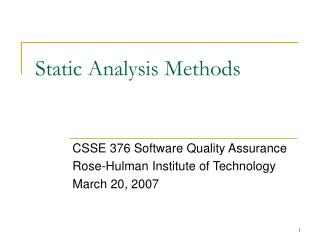DownloadDownload PresentationStatic Analysis Methods

# Static Analysis Methods

Télécharger la présentation## Static Analysis Methods

- - - - - - - - - - - - - - - - - - - - - - - - - - - E N D - - - - - - - - - - - - - - - - - - - - - - - - - - -
##### Presentation Transcript

1. Static Analysis Methods CSSE 376 Software Quality Assurance Rose-Hulman Institute of Technology March 20, 2007

2. Outline • Cyclomatic complexity • Formal verification • Symbolic execution

3. Cyclomatic Complexity • Measure of the complexity of a function • Defines a minimum number of tests to run • Vg = # regions of planar flow graph • Vg = E - N + 2 (edges - nodes + 2) • Vg = P + 1 (predicates + 1)

4. Example of Cyclomatic Complexity (Corrected 3/29/07)

5. Using Cyclomatic Complexity • Vg is the number of independent paths through the function • Each path should be tested at least once • Vg is also a measure of complexity: a large value is a warning that the code may need extra testing or should be rewritten

6. Cartoon of the Day

7. Formal Verification • Compare implementation to a formal specification • Use rules like Assignment Axiom: /* P(E) */ V = E; /* P(V) */

8. Automated Support for Formal Verification • Simple rules like Assignment Axiom can be automated. • Some formulas will need to be simplified for tools to recognize similarity. • Some theorems may need to be proved to complete the verification

9. Symbolic Execution • Execution of code using symbolic values instead of real data • Compare symbolic values with expected values (results) • Path Condition: Condition on input variables under which this path is executed

10. Example Program Path Condition 1: read c; True 2: r = 0; True 3: while (c > 0) { True 4: r = r + a; c0 > 0 5: c = c - 1; } c0 > 0 6: print r; c <= 0